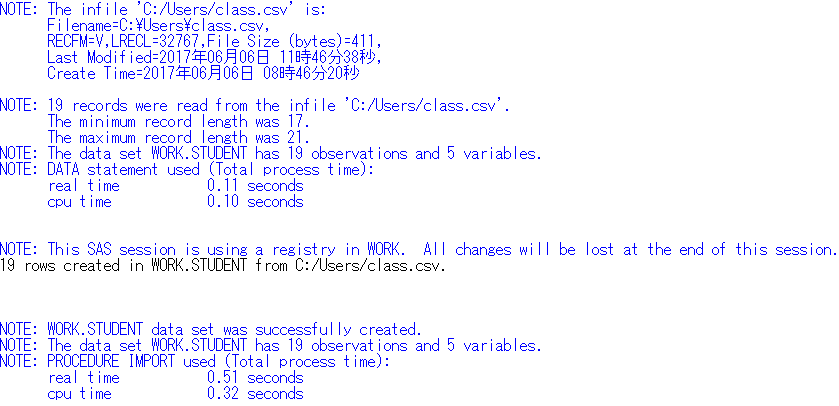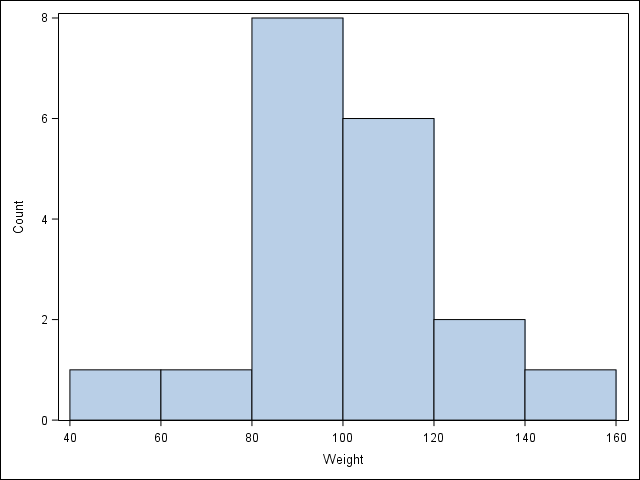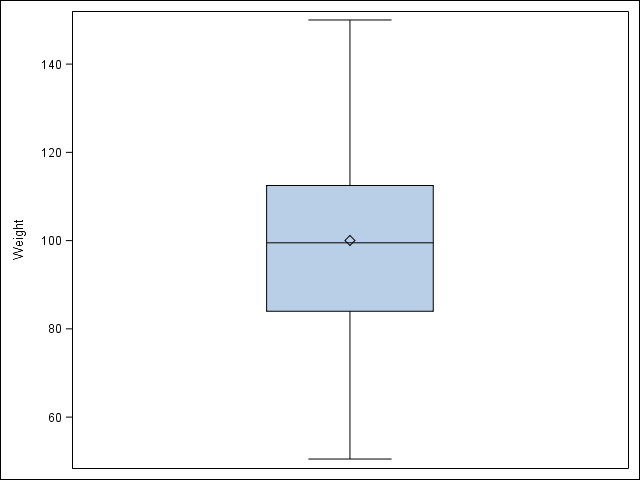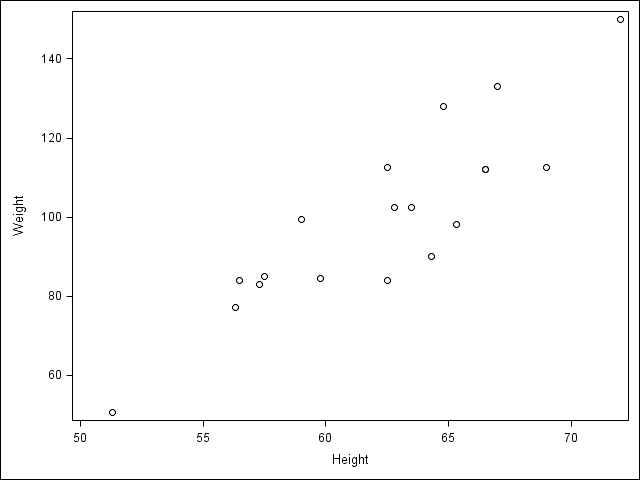# SAS Tutorial

Welcome to the SAS tutorial version of Data Science Rosetta Stone. Before beginning this tutorial, please check to make sure you have SAS 14.2 installed (this is not required, but this was the release used to generate the following examples). SAS Enterprise Miner Workstation 14.2 was used to produce some of the following results.

You also may need to insure that your SAS environment is connected with an R environment so that the R code that SAS calls at the end of this tutorial from the IML Procedure runs successfully.

Note: In SAS,

``````*  This is a single line comment ;
/* This is a paragraph
comment */``````

Now let’s get started!

# 1 Reading in Data and Basic Statistical Functions

## 1.1 Read in the data.

The IMPORT Procedure is useful for reading in SAS data sets of a variety of different types.

### a) Read the data in as a .csv file.

``````proc import out = student
datafile = 'C:/Users/class.csv'
dbms = csv replace;
getnames = yes;
run;``````

### b) Read the data in as a .xls file.

``````proc import out = student_xls
datafile = 'C:/Users/class.xls'
dbms = xls replace;
getnames = yes;
run;``````

### c) Read the data in as a .json file.

There is more code involved in reading a .json file into SAS so that all the format is correct, however we will not at this time dive into the explanation for all this code, but please see the links below.

``````data student_json;
INFILE 'C:/Users/class.json' LRECL  = 3456677  TRUNCOVER SCANOVER
dsd
dlm=",}";
INPUT
@'"Name":' Name : \$12.
@'"Sex":' Sex : \$2.
@'"Age":' Age :
@'"Height":' Height :
@'"Weight":' Weight :
@@;
run;``````

DATA step: infile & input statements

## 1.2 Find the dimensions of the data set.

The shape of a SAS data set is available by running the IMPORT Procedure and looking at the notes in the log file.

``````proc import out = student
datafile = 'C:/Users/class.csv'
dbms = csv replace;
getnames = yes;
run;``````## 1.3 Find basic information about the data set.

The CONTENTS procedure prints information about a SAS data set.

``````proc contents data = student;
run;``````
``````                          The CONTENTS Procedure

Data Set Name        WORK.STUDENT                  Observations          19
Member Type          DATA                          Variables             5
Engine               V9                            Indexes               0
Created              07/05/2017 13:49:31           Observation Length    32
Protection                                         Compressed            NO
Data Set Type                                      Sorted                NO
Label
Data Representation  WINDOWS_64
Encoding             wlatin1  Western (Windows)

Alphabetic List of Variables and Attributes

#    Variable    Type    Len    Format     Informat

3    Age         Num       8    BEST12.    BEST32.
4    Height      Num       8    BEST12.    BEST32.
1    Name        Char      7    \$7.        \$7.
2    Sex         Char      1    \$1.        \$1.
5    Weight      Num       8    BEST12.    BEST32. ``````

## 1.4 Look at the first 5 (last 5) observations.

The PRINT procedure prints a SAS data set, according to the specifications and options provided.

``````/* obs= option tells SAS how many observations to print, starting
with the first observation */
proc print data = student (obs=5);
run;``````
``````   Obs    Name       Sex             Age          Height          Weight

1    Alfred      M               14              69           112.5
2    Alice       F               13            56.5              84
3    Barbara     F               13            65.3              98
4    Carol       F               14            62.8           102.5
5    Henry       M               14            63.5           102.5``````

``````/* print the last 5 observations */
proc print data = student (firstobs=15);
run; ``````
``````   Obs    Name       Sex             Age          Height          Weight

15    Philip      M               16              72             150
16    Robert      M               12            64.8             128
17    Ronald      M               15              67             133
18    Thomas      M               11            57.5              85
19    William     M               15            66.5             112``````

## 1.5 Calculate means of numeric variables.

The MEANS procedure prints the means of all numeric variables of a SAS data set, as well as other descriptive statistics.

``````proc means data = student mean;
run;``````
``````                            The MEANS Procedure

Variable            Mean
------------------------
Age           13.3157895
Height        62.3368421
Weight       100.0263158
------------------------``````

## 1.6 Compute summary statistics of the data set.

Summary statistics of a SAS data set are available by running the MEANS procedure and specifying statistics to return.

``````/* SAS uses a different method than Python and R to compute
quartiles, but the method in each language can be changed */
/* maxdec= option tells SAS to print at most 2 numbers behind
the decimal point */
proc means data = student min q1 median mean q3 max n maxdec=2;
run;``````
``````                            The MEANS Procedure

Lower
Variable         Minimum        Quartile          Median            Mean
------------------------------------------------------------------------
Age                11.00           12.00           13.00           13.32
Height             51.30           57.50           62.80           62.34
Weight             50.50           84.00           99.50          100.03
------------------------------------------------------------------------

Upper
Variable        Quartile         Maximum     N
----------------------------------------------
Age                15.00           16.00    19
Height             66.50           72.00    19
Weight            112.50          150.00    19
----------------------------------------------``````

## 1.7 Descriptive statistics functions applied to columns of the data set.

``````/* The var statement tells SAS which variable to use for the
procedure */
proc means data = student stddev sum n max min median maxdec=2;
var Weight;
run;``````
``````                            The MEANS Procedure

Analysis Variable : Weight

Std Dev           Sum   N       Maximum       Minimum        Median
------------------------------------------------------------------------
22.77       1900.50  19        150.00         50.50         99.50
------------------------------------------------------------------------``````

## 1.8 Produce a one-way table to describe the frequency of a variable.

The FREQ procedure prints the frequency of categorical or discrete variables of a SAS data set.

### a) Produce a one-way table of a discrete variable.

``````proc freq data = student;
tables Age / nopercent norow nocol;
run;``````
``````                            The FREQ Procedure

Cumulative
Age    Frequency     Frequency
------------------------------
11           2             2
12           5             7
13           3            10
14           4            14
15           4            18
16           1            19 ``````

### b) Produce a one-way table of a categorical variable.

``````proc freq data = student;
tables Sex / nopercent norow nocol;
run;``````
``````                            The FREQ Procedure

Cumulative
Sex    Frequency     Frequency
------------------------------
F             9             9
M            10            19 ``````

The tables statement allows you to specify multiple variables at once, separated only by a space, so both of these tables could have been created with one FREQ procedure call. The options on the tables statement (nopercent norow nocol) prevent SAS from printing percents in the table, which are printed by default.

## 1.9 Produce a two-way table to visualize the frequency of two categorical (or discrete) variables.

``````/* The "*" between two variables on the tables statement
indicates to produce a two-way table of the two variables */
proc freq data = student;
tables Age*Sex / nopercent norow nocol;
run;``````
``````                            The FREQ Procedure

Table of Age by Sex

Age       Sex

Frequency|F       |M       |  Total
---------+--------+--------+
11 |      1 |      1 |      2
---------+--------+--------+
12 |      2 |      3 |      5
---------+--------+--------+
13 |      2 |      1 |      3
---------+--------+--------+
14 |      2 |      2 |      4
---------+--------+--------+
15 |      2 |      2 |      4
---------+--------+--------+
16 |      0 |      1 |      1
---------+--------+--------+
Total           9       10       19``````

FREQ Procedure

## 1.10 Select a subset of the data that meets a certain criterion.

The SAS DATA step is used for all things data manipulation and in Section 2 we will explore it further.

``````data females;
set student;
where Sex = "F";
run;
proc print data = females(obs=5);
run; ``````
``````   Obs    Name       Sex             Age          Height          Weight

1     Alice       F               13            56.5              84
2     Barbara     F               13            65.3              98
3     Carol       F               14            62.8           102.5
4     Jane        F               12            59.8            84.5
5     Janet       F               15            62.5           112.5``````

DATA step: set & where statements

## 1.11 Determine the correlation between two continuous variables.

``````/* The nosimple option reduces the output of this procedure */
proc corr data = student pearson nosimple;
var Height Weight;
run; ``````
``````                            The CORR Procedure

2  Variables:    Height   Weight

Pearson Correlation Coefficients, N = 19
Prob > |r| under H0: Rho=0

Height        Weight

Height       1.00000       0.87779
<.0001

Weight       0.87779       1.00000
<.0001              ``````

CORR Procedure

# 2 Basic Graphing and Plotting Functions

The SGPLOT procedure is a very useful SAS procedure for producing plots from data. For more information on other statements within the SGPLOT procedure, please see the Appendix Section 2.

## 2.1 Visualize a single continuous variable by producing a histogram.

``````proc sgplot data = student;
histogram weight / binwidth=20 binstart=40 scale=count;
xaxis values=(40 to 160 by 20);
run;``````## 2.2 Visualize a single continuous variable by producing a boxplot.

``````/* SAS automatically prints the mean on the boxplot */
proc sgplot data = student;
vbox Weight;
run;``````## 2.3 Visualize two continuous variables by producing a scatterplot.

``````/* Notice here you specify the y variable followed by the x variable */
proc sgscatter data = student;
plot Weight * Height;
run; ``````SGSCATTER Procedure